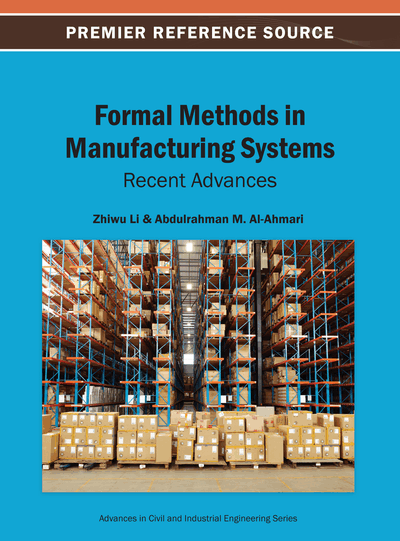# Design of Optimized Petri Net Supervisors for Flexible Manufacture Systems Based on Elementary Siphons

Mingming Yan (University of Electronic Science and Technology of China, China)
Copyright: © 2013 |Pages: 21
DOI: 10.4018/978-1-4666-4034-4.ch013
OnDemand PDF Download:
Available
\$37.50
No Current Special Offers

## Abstract

This chapter focuses on the deadlock prevention problems in Flexible Manufacturing Systems (FMS), and the major target is to design more excellent controllers that lead to a more permissive supervisor by adding a smaller number of monitors and arcs than the existing ones in the literature for the design of liveness-enforcing Petri net supervisors. The authors distinguish siphons in a Petri net model by elementary and dependent ones. For each elementary siphon, a monitor is added to the plant model such that it is invariant-controlled without generating emptiable control-induced siphons, and the controllability of a dependent siphon is ensured by changing the control depth variables of its related elementary siphons. Hence, a structurally simple Petri net supervisor is achieved. Based on the previous work, this chapter explores two optimized deadlock prevention approaches based on elementary siphons that can achieve the same control purpose and have more excellent performance.
Chapter Preview
Top

## 2. Preliminaries

### 2.1. Petri Nets

The following theories are from (Ezpeleta, Colom & Martinez, 1995; Banaszak & Krogh, 1990; Barkaoui & Pradat-Peyre, 1996; Chu & Xie, 1997; Hsien & Chang, 1994; Zhou, Dicesare & Desrochers, 1992; Zhou & Dicesare, 1991; and Zhou, Dicesare & Rudolph, 1992).

A Petri net is a four-tuple N=(P, T, F, W) where P and T are finite and non-empty. P is the set of places and T is the set of transitions with PTΦ ; and PT=Φ. F⊆(P×T)∪(T×P) is called the flow relation or the set of directed arcs. W:FN is a mapping that assigns a weight to any arc, where N={0, 1, 2, …}. N=(P, T, F, W) is ordinary, denoted as N=(P, T, F), if ∀ fF, W(f)=1. Unless otherwise stated, we consider only ordinary Petri nets in this chapter. Incidence matrix (N) of net N is a |P|×|T| integer matrix and (N)(p, t)=W(t, p)-W(p, t). The preset of a node xP×T is defined as x={yPT∣(y, x)∈F}. While the postset of a node xPT is defined as x={yPT∣(x, y)∈F}. This notation can be extended to a set of nodes as follows: given XPT, X=∪xXx and X=∪xX x. A marking is a mapping W:F→ N.

The pair (N, M0) is called a marked Petri net or a net system. The set of markings reachable from M in N is denoted as R(N, M). (N, M0) is bounded if ∃ k∈N, ∀MR(N, M0), ∀ pP, M(p)≤k holds. A transition tT is enabled under M, denoted by M(t〉, if ∀pt: M(p)≥1. A transition tT is live under M0 if ∀MR(N, M0), ∃ M′∈R(N, M), M′(t> holds. (N, M0) is deadlock-free if ∀ MR(N, M0), ∃ tT, M(t〉 holds. (N, M0) is live if ∀ tT, t is live under M0.

A string x1, x2, …, xn is called a path of N if and only if ∀ i∈{1,2,..., n-1}: xi+1xi, where ∀ x∈{x1, x2, …, xn}, xPT. A simple path from x1 to xn is denoted by SP(x1, xn). Petri net N is called a state machine if ∀ tT, |t|=|t|=1. A state machine component N′ = (P′, T′, F′, W′) of a Petri net N=(P, T, F, W) is a state machine and is a subnet of N consisting of places in P′, their presets and postsets, and related arcs. A Petri net is said to be state machine decomposable if it is covered by state machine components.

A nonempty set SP is a siphon if SS holds. A siphon is minimal if there is no siphon contained in S as a proper subset. A minimal siphon S is called a strict minimal siphon if SS.

A P-vector is a column vector I: PZ indexed by P, where Z is the set of integers. I is a P-invariant (place invariant) if I≠0 and I T(N)=0T. P-invariant I is said to be a P-semiflow if every element of I is non-negative. ||I||={pPI(p)≠0} is called the support of I. If I is a P-invariant of (N, M0) then ∀ MR(N, M0): ITM=ITM0. Siphon S is controlled by P-invariant I under M0 if ITM0 >0 and {pPI(p)>0}⊆S}. Such a siphon is called invariant-controlled.

## Complete Chapter List

Search this Book:
Reset